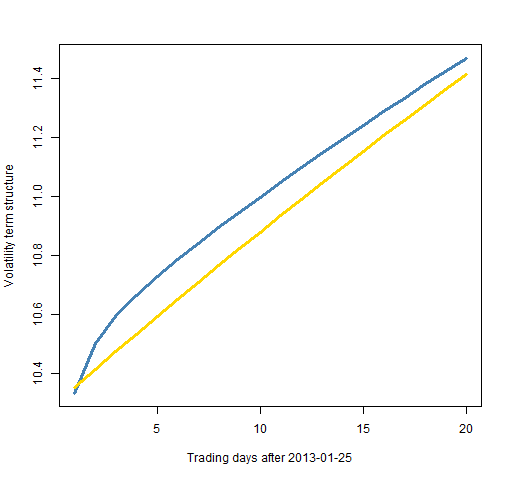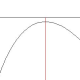# The components garch model in the rugarch package

How to fit and use the components model.

## Previously

Related posts are:

## The model

The components model (created by Engle and Lee) generally works better than the more common garch(1,1) model.  Some hints about why it is better are in “3 realms of garch modelling”.

Figure 1 shows predictions of volatility for each day 20 days into the future for two models fit on the S&P 500.  The components model has more nuanced predictions of volatility.

Figure 1: Volatility predictions for each day with the components model (blue) and the garch(1,1) with variance targeting (gold).It is quite possible that this is not the question you would really like answered.  Often what you care about is the average volatility from now until the appointed day.  This is sometimes referred to as the term structure — Figure 2 shows this.

Figure 2: Predicted volatility term structure for the S&P 500 using the components model (blue) and the garch(1,1) with variance targeting (gold).The code to create these plots is shown below.

## R code

The `rugarch` package allows the option of fitting the components model.

### An error

We’ll get to how to do things presently, but first here is a problem I ran into:

```> spx.garct <- ugarchfit(comtspec, tail(spxret, 2000))
Error in .hessian2sidedcpp(f, ipars[estidx, 1], arglist = arglist) :
SET_VECTOR_ELT() can only be applied to a 'list', not a 'symbol'
> packageVersion('rugarch')
 ‘1.0.16’```

I knew what to do after seeing this error because I read the r-sig-finance mailing list.  The hypothesis was that changes to `Rcpp` and relatives probably caused this problem.  So updating those packages should do the trick.

```> detach(package:Rcpp, unload=TRUE)
Error: package ‘Rcpp’ is required by ‘rugarch’ so will not be detached

After unloading the packages, I updated the `Rcpp` and `RcppArmadillo` packages.  Things still didn’t work right, so I did the same exercise of updating `numDeriv` as well.  Then I had different weird behavior.

It turned out there was still a problem with the installation of `Rcpp`.  After installing `Rcpp` once more, there was success.

If I hadn’t seen the r-sig-finance message with a similar error, then my strategy probably would have included doing a web search on a portion of the error message.  In this case the r-sig-finance thread would have shown up.

### Onwards

The components model is called `"csGARCH"` by `rugarch` and the two extra parameters are called `eta11` (ρ in my notation) and `eta21` (φ).  A specification of it with a t distribution and just a constant mean is:

```comtspec <- ugarchspec(mean.model=list(
armaOrder=c(0,0)), distribution="std",
variance.model=list(model="csGARCH"))```

This specification is used like:

```> spx.garct <- ugarchfit(comtspec, tail(spxret, 2000))
Warning message:
In .makefitmodel(garchmodel = "csGARCH", f = .csgarchLLH, T = T,  :
NaNs produced```

The warning message seems to be about:

```> spx.garct@fit\$condH
 NaN```

I’m not sure what this is, but I suspect it isn’t a tragedy that we don’t have it.

The estimated coefficients and half-life are:

```> coef(spx.garct)
mu        omega       alpha1        beta1
7.524949e-04 1.245354e-06 2.892064e-08 8.937168e-02
eta11        eta21        shape
9.918261e-01 8.934750e-02 6.010112e+00
> halflife(spx.garct)
Transitory  Permanent
0.2870233 84.4534694```

Variance targeting seems not to be implemented (yet).

The specification for the garch(1,1) model with variance targeting is:

```tvtspec <- ugarchspec(mean.model=list(
armaOrder=c(0,0)), distribution="std",
variance.model=list(model="sGARCH",
variance.targeting=TRUE))```

### Prediction

Once the models are fit, you can predict.

```spx.ctvolfore <- ugarchforecast(spx.garct,
100 * sqrt(252)

spx.tvtvolfore <- ugarchforecast(spx.gartvt,
100 * sqrt(252)```

The structure of the forecast output is not exactly intuitive, but the `str` function is your friend.

Once we have the predicted volatility in hand, we can plot it.  The function to produce Figure 1 is:

```  function (filename = "dailyvolpred.png")
{
# placed in public domain 2012 by Burns Statistics

if(length(filename)) {
png(file=filename, width=512)
par(mar=c(5,4, 3, 2) + .1)
}
plot(1:20, spx.tvtvolfore, type='l', lwd=3,
col="gold",
ylab="Daily volatility prediction")
lines(1:20, spx.ctvolfore, type='l',
col='steelblue', lwd=3)
if(length(filename)) {
dev.off()
}
}```

The function for Figure 2 is:

```  function (filename = "volpredterm.png")
{
# placed in public domain 2012 by Burns Statistics

if(length(filename)) {
png(file=filename, width=512)
par(mar=c(5,4, 3, 2) + .1)
}
plot(1:20, sqrt(cumsum(spx.ctvolfore^2)/(1:20)),
type='l', lwd=3, col="steelblue",
ylab="Volatility term structure")
lines(1:20, sqrt(cumsum(spx.tvtvolfore^2)/(1:20)),
type='l', col='gold', lwd=3)
if(length(filename)) {
dev.off()
}
}```

The value for the `mar` parameter was intended to be:

`c(5, 4, 0, 2) + .1`

but somehow escaped notice until I was too lazy to change it.

This entry was posted in Quant finance, R language and tagged . Bookmark the permalink.

### 9 Responses to The components garch model in the rugarch package

1.Terry Auld says:

Hi
I’ve played with rugarch quite a bit, including the component garch model. Generally speaking, the results agree with EViews 7’s estimations.

In my experience, the NaNs problem only shows up with particular series (and not all that often). Why this is so, I don’t know.

•Pat says:

Terry,

Thanks for the information.

2.Jesper Hybel Pedersen says:

I had the same problem estimating an sGARCH(2,1). And generally any GARCH of order above (1,1) in variancemodel, so I tried to use 10 years of dayli returns instead of 8.. and that worked. Now I was able to estimate up to an order of (2,2) in the variancemodel but with higher orders same problem reappeared.

3.Keiran says:

This is a typically insightful post on an interesting piece of financial modelling. The mini-tutorial on dealing with obscure R errors however is priceless. Thank you.

4.AJ says:

I don’t get the sqrt(252)

5.Jayant says:

I am running around 520 models with 500 observations in each of the models, using the rugarch package. While all my models are successfully converging some of the have NaN value in CondH. I am not sure if I can safely use that model fits for further analysis? I did try to optimize some of those models in Eviews and Matlab and I was successfully able to do it with any errors. The parameters were also more or less similar. Can you please elaborate more on ConH ?